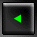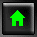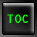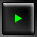##Appendix A Operator Precedence

It is important to understand that operators have a precedence, but it is not essential to memorize the precedence.

New Term: Precedence is the order in which a program performs the operations in a formula. If one operator has precedence over another operator, it is evaluated first.

Higher precedence operators "bind tighter" than lower precedence operators; thus, higher precedence operators are evaluated first. The lower the rank in the following chart, the higher the precedence.

Table A.1. Operator Precedence.
 Rank Name Operator 1 scope resolution :: 2 member selection, subscripting, . -> function calls, postfix increment () and decrement ++ -- 3 sizeof, prefix increment and decrement, ++ -- complement, and, not, unary minus and plus, ^ ! address of and dereference, new, new[], delete, - + delete[], casting, sizeof(), & * () 4 member selection for pointer .* ->* 5 multiply, divide, modulo * / % 6 add, subtract + - 7 shift << >> 8 inequality relational < <= > >= 9 equality, inequality == != 10 bitwise AND & 11 bitwise exclusive OR ^ 12 bitwise OR | 13 logical AND && 14 logical OR || 15 conditional ?: 16 assignment operators = *= /= %= += -= <<= >>= &= |= ^= 17 throw operator throw 18 comma ,